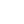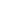# Python分析淘宝4403条数据，终于找到最适合夏天穿的大裤衩

1.国内哪些地方的大裤衩卖的比较好？

2.大裤衩市场价格是怎样的？

3.哪些店铺大裤衩销量较高？

4.在售的大裤衩具有哪些特点？

### 一、数据获取`````` def main():
browser.get('https://www.taobao.com/')
page = search_product(key_word)
print(page)
get_data()
page_num = 1
while int(page) != page_num:
print("-" * 100)
print("正在爬取第{}页大裤衩数据".format(page_num + 1))
browser.get('https://s.taobao.com/search?q={}&s={}'.format(key_word, page_num*44))
browser.implicitly_wait(10)
get_data()
page_num += 1
print("大裤衩数据抓取完成")

if __name__ == '__main__':
key_word = "大裤衩 男"
browser = webdriver.Chrome("./chromedriver")
main()``````

### 二、数据清洗

#### 1.导入数据

``````import pandas as pd
import numpy as np
names=['商品名称','商品价格','付款人数','店铺名称','发货地址']) #添加字段名称

df.sample(5) #预览数据``````#### 2.删除重复记录

``df = df.drop_duplicates() ``

#### 3.查看数据信息

``````df.info()
<class 'pandas.core.frame.DataFrame'>
Int64Index: 3835 entries, 0 to 4402
Data columns (total 5 columns):
#   Column  Non-Null Count  Dtype
---  ------  --------------  -----
0   商品名称    3835 non-null   object
1   商品价格    3835 non-null   float64
2   付款人数    3835 non-null   object
3   店铺名称    3835 non-null   object
4   发货地址    3822 non-null   object
dtypes: float64(1), object(4)
memory usage: 179.8+ KB``````

#### 4.缺失值处理

``df.dropna(axis=0, how='any', inplace=True)``

#### 5.商品价格字段处理

``````listBins = [0, 50,100, 150, 200, 500,1000000] #设置切分区域
listLabels = ['50及以下','51-100','101-150','151-200','201-500','500及以上']#设置切分后对应标签
df['价格区间'] = pd.cut(df['商品价格'], bins=listBins, labels=listLabels, include_lowest=True) #利用pd.cut进行数据离散化切分``````

#### 6.发货地址字段处理

``````df["省份"] = df["发货地址"].str.split(' ',expand=True)  #expand=True可以把用分割的内容直接分列
#df['省份'] = df['发货地址'].str.split(' ').apply(lambda x:x) #提取省份
df["城市"] = df["发货地址"].str.split(' ',expand=True) #提取城市
df["城市"].fillna(df["省份"], inplace=True) #城市字段空值用省份非空值填充``````

#### 7.付款人数字段处理

``````import re
df['数字'] = [re.findall(r'(\d+\.{0,1}\d*)', i) for i in df['付款人数']]  # 提取数值
df['数字'] = df['数字'].astype('float')  # 转化数值型
df['单位'] = [''.join(re.findall(r'(万)', i)) for i in df['付款人数']]  # 提取单位（万）
df['单位'] = df['单位'].apply(lambda x:10000 if x=='万' else 1)
df['付款人数'] = df['数字'] * df['单位'] # 计算付款人数``````

#### 8.其他处理

``````# 删除多余的列
df.drop(['发货地址', '数字', '单位'], axis=1, inplace=True)
#按商品价格降序
df = df.sort_values(by="商品价格", axis=0, ascending=False)
# 重置索引
df = df.reset_index(drop=True)### 三、数据可视化#### 1.国内哪些地方的大裤衩卖的比较好？#### 2.大裤衩市场价格是怎样的？#### 3.哪些店铺大裤衩销量较高？#### 4.在售的大裤衩具有哪些特点？#### 5.选择合适的大裤衩## 立即试用FineBI，还可获得更多的方案、案例和模板售前咨询：400-811-8890转1投诉建议：173-127-81526海外咨询电话：（+86）0510-66758729400-811-8890转1800049425

400-811-8890转2173-127-81526